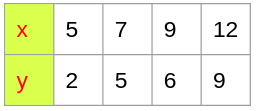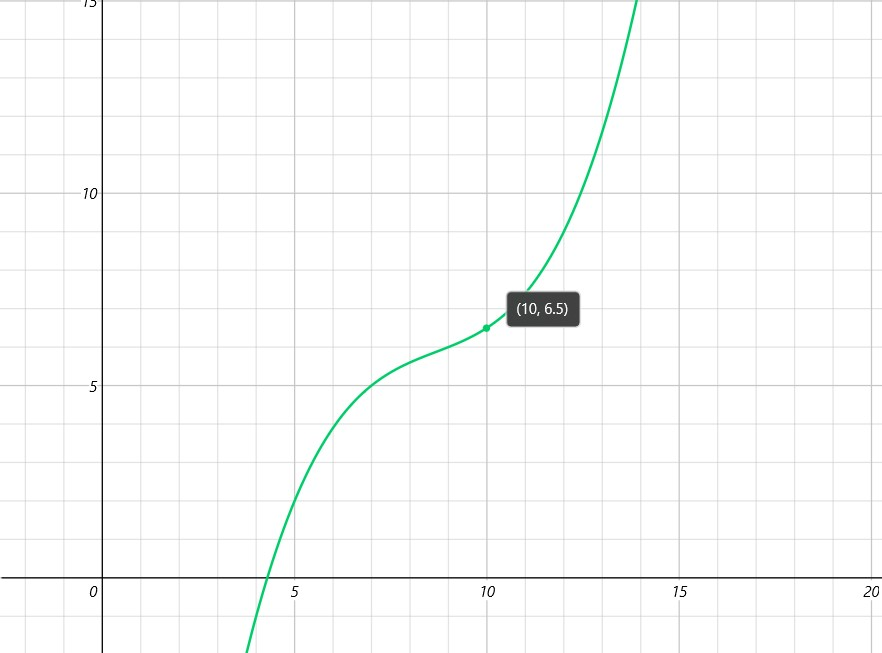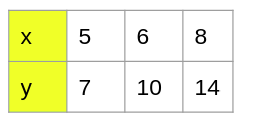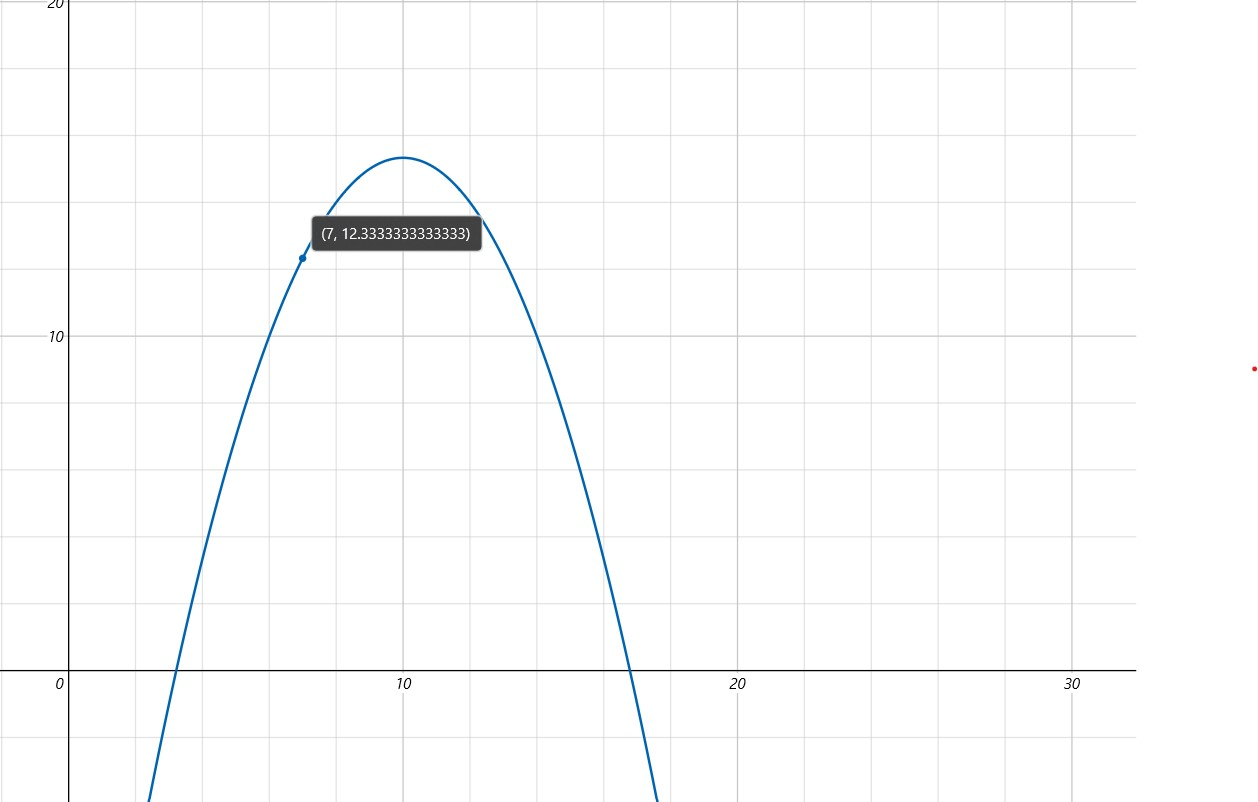Polynomial Interpolation is a method that used in estimating the values between the two data points,to estimate the values ,the data should be available on both sides of the data or at a few specific points .An estimation data between the gap can be made by using the interpolation

There are some methods for finding the Interpolation mentioned below

## Lagrange's Method

Polynomial is the simplest form of an interpolant. we can construct an unique polynomial of degree n that passes through (n+1) distinct data points.$P_n(x) =\sum_{i=0}^{n} y_i l_i(x)$

here n in$P_n(x)$  denotes the degree of the polynomial and$l_i(x) =\frac{x-x_0}{x_i-x_0} \frac{x-x_1}{x_i-x_1}.... \frac{x-x_i-1}{x_i-x_i-1}.\frac{x-x_i+1}{x_i-x_i+1} ....\frac{x-x_n}{x_i-x_n} \newline \newline l_i(x) = \prod_{j=0 , j \neq i}^{n} \frac {x-x_i}{x_i-x_j} , i =0,1,2,3....n$

here$l_i (x) for i = 0,1,2....n$ are called cardinal functions.

now let us consider an example

let n=1 then$P_1(x)=y_0 l_0 + y_1 l_1$ is the interpolant for the straight line

where$l_0 (x) = \frac{x-x_1}{x_0-x_1}$   and$l_1(x)= \frac{x-x_0}{x_1-x_0}$

now let us consider n=2 ,then the equation becomes$P_2(x) = y_0 l_0(x) +y_ 1 l_1(x) +y_2 l_2(x)$ is the interpolant for the parabola (polynomial of degree 2)

here$l_0(x) = \frac{(x-x_1)(x-x_2)}{x_0-x_1)(x_0-x_2)} \newline$ ,$l_1(x) = \frac{(x-x_0)(x-x_2)}{x_1-x_0)(x_1-x_2)}$,$l_0(x) = \frac{(x-x_0)(x-x_1)}{x_2-x_0)(x_2-x_1)}$,

Cardinal fucntions are polynomials of degree n and have the property$l_i (x_i)= \Bigg\{ 0 \ if \ i \neq j \hspace{0.5cm} and \hspace{0.5cm} 1 \ if \ \ i =j \Bigg\} =\delta_ij$ ,

here the$\delta_ij$ is known as kronecker delta

Now to verify that the interpolating polynomial passes through the given data points ,we will substitue$x = x_j$ in$p_n(x) = \sum_{i=0}^{n} y_i l_i(x)$ , and use$l_i (x_i)= \Bigg\{ 0 \ if \ i \neq j \hspace{0.5cm} and \hspace{0.5cm} 1 \ if \ \ i =j \Bigg\} =\delta_ij$

then we get ,$P_n(x_j) = \sum_{i=0}^{n} y_i l_i (x_j) = \sum_{i=0}^{n} y_i \delta_ij = y_j$

Example : 1

find$y(10)$ from the following data set given belowIn the above given data set the intervals are unequal .By using the lagrange’s interpolation  we have$x_0 =5 \ x_1=7 \ x_2=9 \ x_3=12 \\ y_0 = 2 \ y_1=5 \ y_2=6 \ y_3=9$,$y = f(x) =\Bigg\{ \frac{(x-x_1) (x-x_2) (x-x_3) }{(x_0-x_1)(x_0-x_2)(x_0-x_3)} * y_0 + \frac{(x-x_0)(x-x_2)(x-x_3)}{(x_1-x_0)(x_1-x_2)(x_1-x_3)} * y_1 \\ \hspace{1cm} + \frac{(x-x_0)(x-x_1)(x-x_3)}{(x_2-x_0)(x-2-x_1)(x_2-x-3)}*y_2 + \frac{(x-x_0)(x-x_1)(x-x_2)}{(x_3-x_0)(x_3-x_1)(x_3-x_2)} * y_3 \Bigg\}$,$f(x) = 2\frac{(x-7) \cdot (x-9) \cdot (x-12)}{(5-7) \cdot (5-9) \cdot (5-12)}+5\frac{(x-5) \cdot (x-9) \cdot (x-12)}{(7-5) \cdot (7-9) \cdot (7-12)}+6\frac{(x-5) \cdot (x-7) \cdot (x-12)}{(9-5) \cdot (9-7) \cdot (9-12)}+9\frac{(x-5) \cdot (x-7) \cdot (x-9)}{(12-5) \cdot (12-7) \cdot (12-9)}$$f(x) = 2\frac{(x-7) \cdot (x-9) \cdot (x-12)}{(-2) \cdot (-4) \cdot (-7)}+5\frac{(x-5) \cdot (x-9) \cdot (x-12)}{2 \cdot (-2) \cdot (-5)}+6\frac{(x-5) \cdot (x-7) \cdot (x-12)}{4 \cdot 2 \cdot (-3)}+9\frac{(x-5) \cdot (x-7) \cdot (x-9)}{7 \cdot 5 \cdot 3}$$f(x) = 2\frac{(x^2-16x+63) \cdot (x-12)}{8 \cdot (-7)}+5\frac{(x^2-14x+45) \cdot (x-12)}{(-4) \cdot (-5)}+6\frac{(x^2-12x+35) \cdot (x-12)}{8 \cdot (-3)}+9\frac{(x^2-12x+35) \cdot (x-9)}{35 \cdot 3}$$f(x) = 2\frac{(x^3-28x^2+255x-756)}{(-56)}+5\frac{(x^3-26x^2+213x-540)}{20}+6\frac{(x^3-24x^2+179x-420)}{(-24)}+9\frac{(x^3-21x^2+143x-315)}{105}$$f(x) = -\frac{1}{28}(x^3-28x^2+255x-756)+\frac{1}{4}(x^3-26x^2+213x-540)-\frac{1}{4}(x^3-24x^2+179x-420)+\frac{3}{35}(x^3-21x^2+143x-315)$$f(x) = (-\frac{1}{28}x^3+x^2-\frac{255}{28}x+27)+(\frac{1}{4}x^3-\frac{13}{2}x^2+\frac{213}{4}x-135)+(-\frac{1}{4}x^3+6x^2-\frac{179}{4}x+105)+(\frac{3}{35}x^3-\frac{9}{5}x^2+\frac{429}{35}x-27)$$f(x) = \frac{1}{20}x^3-\frac{13}{10}x^2+\frac{233}{20}x-30$

Now, we need to find the value of$y(10) as f(10) =y(10)$ ,so put$x = 10$ in the above equation,

on calculating$f(10) =0 ,we get \\ f(10) = \frac{13}{2} =6.5$

Now lets try to solve the problem  by using s2

				
// this is the java code
OrderedPairs data = new SortedOrderedPairs(
new double[]{5,7,9,12},
new double[]{2,5,6,9}
);
LinearInterpolation li = new LinearInterpolation();
UnivariateRealFunction f = li.fit(data);
System.out.println(f.evaluate(10)); // f(2) = 6.5


				
// converted kotlin coee
%use s2
var data01 = SortedOrderedPairs (doubleArrayOf(5,7,9,12),doubleArrayOf (2,5,6,9))
var li : LinearInterpolation = LinearInterpolation()
var f1: UnivariateRealFunction = li.fit(data01)
println(f1.evaluate(10))


				
%use s2
//here let x be the given given value to find the interpolant
var x : Double = 10.0

//defining the variables for the  given data
var x_0 : Double = 5.0
var y_0 : Double = 2.0
var l_0 : Double         //here l_0 be the l(0) as we discuused in the above

var x_1 : Double = 7.0
var y_1 : Double = 5.0
var l_1 : Double             //here l_0 be the l(1)

var x_2 : Double = 9.0
var y_2 : Double = 6.0
var l_2 : Double              //here l_0 be the l(2)

var x_3 : Double = 12.0
var y_3 : Double = 9.0
var l_3 : Double              //here l_0 be the l(3)
//assigning the l(x) values to the variables
l_0= (((x-x_1)*(x-x_2)*(x-x_3))/((x_0-x_1)*(x_0-x_2)*(x_0-x_3)))
l_1= (((x-x_0)*(x-x_2)*(x-x_3))/((x_1-x_0)*(x_1-x_2)*(x_1-x_3)))
l_2= (((x-x_0)*(x-x_1)*(x-x_3))/((x_2-x_0)*(x_2-x_1)*(x_2-x_3)))
l_3= (((x-x_0)*(x-x_1)*(x-x_2))/((x_3-x_0)*(x_3-x_1)*(x_3-x_2)))

var f : Double
f = l_0*y_0 + l_1*y_1 + l_2*y_2 +l_3*y_3

println("The value of y(10) = $f")   Output : The value of y(10) = 6.5 Plot   %use s2 // plotting the above function using JGnuplot val p = JGnuplot(false) p.addPlot("x*x*x*1/20 - 13/10*x*x + 223/20*x -30 ") p.plot()   Output :Example 2 : find the vale of$y(7)$ from the above given datasetIn the above given data set the intervals are unequal .By using the lagrange’s interpolation we have$x_0 =5 \ x_1=6 \ x_2=8 \\ y_0 = 7 \ y_1=10 \ y_2=14$,$f(x) = 7\frac{(x-6) \cdot (x-8)}{(5-6) \cdot (5-8)}+10\frac{(x-5) \cdot (x-8)}{(6-5) \cdot (6-8)}+14\frac{(x-5) \cdot (x-6)}{(8-5) \cdot (8-6)}$$f(x) = 7\frac{(x-6) \cdot (x-8)}{(-1) \cdot (-3)}+10\frac{(x-5) \cdot (x-8)}{1 \cdot (-2)}+14\frac{(x-5) \cdot (x-6)}{3 \cdot 2}$$f(x) = 7\frac{(x^2-14x+48)}{3}+10\frac{(x^2-13x+40)}{(-2)}+14\frac{(x^2-11x+30)}{6}$$f(x) = \frac{7}{3}(x^2-14x+48)-5(x^2-13x+40)+\frac{7}{3}(x^2-11x+30)$$f(x) = (\frac{7}{3}x^2-\frac{98}{3}x+112)+(-5x^2+65x-200)+(\frac{7}{3}x^2-\frac{77}{3}x+70)$$f(x) = -\frac{1}{3}x^2+\frac{20}{3}x-18$ Now, we need to find the value of$y(7) as f(7) =y(7)$ ,so put$x= 7$ in the above equation, on calculating,$f(7) \ we \ get \newline f(7) = 12.334$ . testing Output : The value of y(7) = 12.333333333333332   %use s2 //here let x be the given given value to find the interpolant var x : Double = 7.0 //defining the variables for the given data var x_0 : Double = 5.0 var y_0 : Double = 7.0 var l_0 : Double //here l_0 be the l(0) as we discuused in the above var x_1 : Double = 6.0 var y_1 : Double = 10.0 var l_1 : Double //here l_0 be the l(1) var x_2 : Double = 8.0 var y_2 : Double = 14.0 var l_2 : Double //here l_0 be the l(2) //assigning the l(x) values to the variables l_0= (((x-x_1)*(x-x_2))/((x_0-x_1)*(x_0-x_2))) l_1= (((x-x_0)*(x-x_2))/((x_1-x_0)*(x_1-x_2))) l_2= (((x-x_0)*(x-x_1))/((x_2-x_0)*(x_2-x_1))) var f : Double f = l_0*y_0 + l_1*y_1 + l_2*y_2 println("The value of y(7) =$f")



PLOT

				
%use s2
// plot the above function by using JGnuplot
val p = JGnuplot(false)